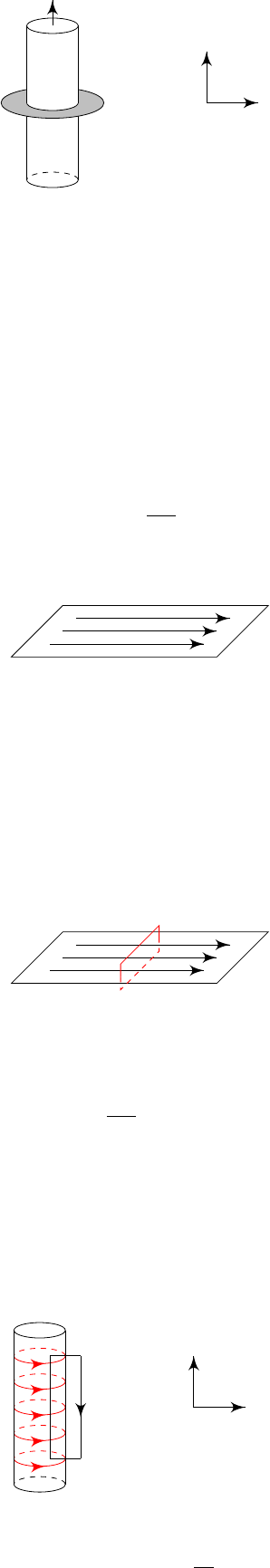3Magnetostatics

IB Electromagnetism3.1 Ampere’s Law
Consider a surface
S
with boundary
C
. Current
J
flows through
S
. We now
integrate the first equation over the surface S to obtain
Z
S
( × B) · dS =
I
C
B · dr = µ
0
Z
S
J · dS.
So
Law (Ampere’s law).
I
C
B · dr = µ
0
I,
where I is the current through the surface.
Example
(A long straight wire)
.
A wire is a cylinder with current
I
flowing
through it.
We use cylindrical polar coordinates (
r, ϕ, z
), where
z
is along the direction
of the current, and r points in the radial direction.
I
S
z
r
By symmetry, the magnetic field can only depend on the radius, and must lie
in the
x, y
plane. Since we require that
· B
= 0, we cannot have a radial
component. So the general form is
B(r) = B(r)
ˆ
ϕ.
To find
B
(
r
), we integrate over a disc that cuts through the wire horizontally.
We have
I
C
B · dr = B(r)
Z
2π
0
r dϕ = 2πrB(r)
By Ampere’s law, we have
2πrB(r) = µ
0
I.
So
B(r) =
µ
0
I
2πr
ˆ
ϕ.
Example
(Surface current)
.
Consider the plane
z
= 0 with surface current
density k (i.e. current per unit length).
Take the x-direction to be the direction of the current, and the
z
direction to be
the normal to the plane.
We imagine this situation by considering this to be infinitely many copies of
the above wire situation. Then the magnetic fields must point in the
y
-direction.
By symmetry, we must have
B = B(z)
ˆ
y,
with B(z) = B(z).
Consider a vertical rectangular loop of length L through the surface
L
Then
I
C
B · dr = LB(z) LB(z) = µ
0
kL
So
B(z) =
µ
0
k
2
for z > 0.
Similar to the electrostatic case, the magnetic field is constant, and the part
parallel to the surface is discontinuous across the plane. This is a general result,
i.e. across any surface,
ˆ
n × B
+
ˆ
n × B
= µ
0
k.
Example
(Solenoid)
.
A solenoid is a cylindrical surface current, usually made
by wrapping a wire around a cylinder.
z
r
C
We use cylindrical polar coordinates with
z
in the direction of the extension of
the cylinder. By symmetry, B = B(r)
ˆ
z.
Away from the cylinder,
× B
= 0. So
B
r
= 0, which means that
B
(
r
)
is constant outside. Since we know that
B
=
0
at infinity,
B
=
0
everywhere
outside the cylinder.
To compute
B
inside, use Ampere’s law with a curve
C
. Note that only the
vertical part (say of length
L
) inside the cylinder contributes to the integral.
Then
I
C
B · dr = BL = µ
o
INL.
where
N
is the number of wires per unit length and
I
is the current in each wire
(so INL is the total amount of current through the wires).
So
B = µ
0
IN.